#### 相似文章# 外汇交易ABC

MetaTrader 4示例 | 13 二月 2008, 12:245 3780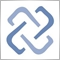### 标准份额大小 - 标准手数

EURO= k*USD
k 表示 EURUSD的交换汇率;
100 000 (k*USD) = X USD => X= k*100 000;

`MarketInfo(Symbol(), MODE_LOTSIZE);`

`MarketInfo("EURUSD",  MODE_LOTSIZE);`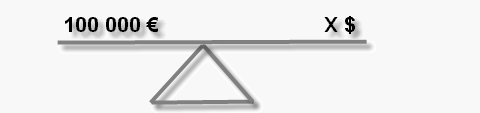### 保证金计算

MarketInfo("GBPJPY", MODE_LOTSIZE) 给出的值 70 000 (LS).
AccountCurrency() 函数返回 "EUR" (AC).
AccountLeverage() 函数返回50 (AL).

M = 70000 GBP / AL = 70 000*GBPEUR / AL = 70000 / EURGBP / AL.

M = 70000 / 0.6786 / 50 = 2063.07 EURO.

M = 70000 / 0.6786 / 10 = 10315.36.

### 点数 - 最小价格改变

`MarketInfo(Symbol(), MODE_DIGITS);`

`MarketInfo("GBPJPY", MODE_DIGITS);`

`MarketInfo(Symbol(), MODE_POINT);`

`MarketInfo(Symbol(), MODE_POINT); and MarketInfo(Symbol(), MODE_TICKSIZE);`

### 在货币存款中的价格点

`MarketInfo(Symbol(), MODE_TICKVALUE);`
`在货币存款中返回点值。必须要注意的是对于货币对点值将会改变，第二个值不是货币存款。`

### 每个标准手的保证金

`MarketInfo(Symbol(), MODE_MARGINREQUIRED);`

### 以上范例的函数使用

```//+------------------------------------------------------------------+
//|                                          SimplySpecification.mq4 |
//|                                       https://www.metaquotes.net/ |
//+------------------------------------------------------------------+
string SymbolsArray = {"","USDCHF","GBPUSD","EURUSD","USDJPY",
//+------------------------------------------------------------------+
//| string SymbolByNumber                                            |
//+------------------------------------------------------------------+
string GetSymbolString(int Number)
{
//----
string res = "";
res = SymbolsArray[Number];
//----
return(res);
}
//+------------------------------------------------------------------+
//| A very simple function to calculate margin for Forex symbols.    |
//| It automatically calculates in the account's base currency and   |
//| does not work for complicated rates that do not have direct      |
//| recalculation into the trade account's base currency.            |
//+------------------------------------------------------------------+
double MarginCalculate(string symbol, double volume)
{
string first    = StringSubstr(symbol,0,3); // the first symbol, for example,  EUR
string second   = StringSubstr(symbol,3,3); // the second symbol, for example, USD
string currency = AccountCurrency();        // deposit currency, for example,  USD
double leverage = AccountLeverage();        // leverage, for example,          100
// contract size, for example, 100000
double contract = MarketInfo(symbol, MODE_LOTSIZE);
double bid      = MarketInfo(symbol, MODE_BID);      // Bid price
//---- allow only standard forex symbols like XXXYYY
if(StringLen(symbol) != 6)
{
Print("MarginCalculate: '",symbol,"' must be standard forex symbol XXXYYY");
return(0.0);
}
//---- check for data availability
if(bid <= 0 || contract <= 0)
{
Print("MarginCalculate: no market information for '",symbol,"'");
return(0.0);
}
//---- check the simplest variations - without cross currencies
if(first == currency)
return(contract*volume / leverage);           // USDxxx
if(second == currency)
return(contract*bid*volume / leverage);       // xxxUSD
//---- check normal cross currencies, search for direct conversion through deposit currency
string base = currency + first;                   // USDxxx
if(MarketInfo(base, MODE_BID) > 0)
return(contract / MarketInfo(base, MODE_BID)*volume / leverage);
//---- try vice versa
base = first + currency;                          // xxxUSD
if(MarketInfo(base, MODE_BID) > 0)
return(contract*MarketInfo(base, MODE_BID)*volume / leverage);
//---- direct conversion is impossible
Print("MarginCalculate: can not convert '",symbol,"'");
return(0.0);
}
//+------------------------------------------------------------------+
//| script program start function                                    |
//+------------------------------------------------------------------+
int start()
{
//----
for(int i = 1; i < 13; i++)
{
"  MODE_TICKVALUE=",MarketInfo(GetSymbolString(i),MODE_TICKVALUE),
", MODE_TICKSIZE=",MarketInfo(GetSymbolString(i),MODE_TICKSIZE),
" MODE_MARGINREQUIRED=",MarketInfo(GetSymbolString(i),MODE_MARGINREQUIRED));
}
//----
return(0);
}
//+------------------------------------------------------------------+```

*大多数情况下，货币对的基础货币是在货币对的第一位，当然，也存在不同的服务器设定。

#### 该作者的其他文章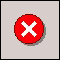错误146 ("交易作业忙") 和如何处理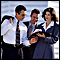MetaTrader 4 客户端的秘密：指标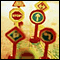MetaTrader 4中的测试特性和限定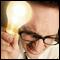使用 MetaTrader 4客户端初学者的常见错误